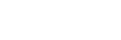# Fake_News_Passive-Aggressive _Classifier_KIT

by souravbanerjee0009

1.The model I've chosen to use is the Passive-Aggressive (PA) Classifier (see original paper here). In essence, the PA classifier is an algorithm that only updates its weights ("aggressive" action) when it encounters examples for which its predictions are wrong, but otherwise remains unchanged ("passive" action). 2.Passive Aggressive Classifier belongs to the category of online learning algorithms in machine learning. It works by responding as passive for correct classifications and responding as aggressive for any miscalculation. 3.It contains 9 libraries, some of the libraries you already know such as pandas, numpy, sklearn. 4.Basic set-up libraries for our models :- i) import os ii) import numpy as np ii) import pandas as pd 5. ML toolkits :- i) from sklearn.model_selection import train_test_split ii) from sklearn.feature_extraction.text import TfidfVectorizer iii) from sklearn.metrics import accuracy_score, confusion_matrix, f1_score iv) from sklearn.utils.extmath import density V ) from sklearn.pipeline import make_pipeline 6.Data visualization :- i) import matplotlib.pyplot as plt ii) import seaborn as sns 7. I have take the data from the internet for Fake_News analysis(Kaggle), you can directly link the Kaggle with Jupiter in order to reduce the time.
• © 2022 Open Weaver Inc.